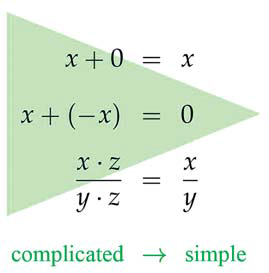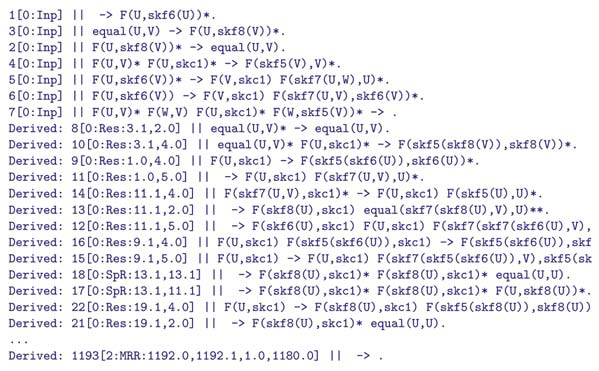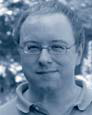# Automated Deduction

## Automated Deduction

In order to guarantee that a piece of hardware or software is working correctly, it must be verified – i.e., its correctness must be proven formally. Proving correctness means that one has to check whether certain properties of the system follow from other properties of the system that are already known. The question of how to use computer programs for such proof tasks has been an important research topic for many decades. Ever since the fundamental theoretic results of Gödel and Turing at the beginning of the twentieth century, it has been known that not everything that is true in a mathematical sense is actually provable, and that not everything that is provable is automatically provable. Correspondingly, deduction systems differ significantly in their expressiveness and properties. For example, decision procedures are specialized for a certain type of data (e.g., real numbers) and are guaranteed to detect the correctness or incorrectness of a statement within this area. Automatic theorem provers for so-called first-order logic can handle any data types defined in a program. For such provers, it is guaranteed only that a proof will be found if one exists; if none exists, the prover may continue searching without success, possibly forever. Even more complicated problems can be handled using interactive provers; these provers, however, only work with user assistance and without any guarantee of completeness.

How does a theorem prover for first-order logic work? It is not difficult to write a program that correctly derives new formulas from given formulas. A logically correct derivation is, however, not necessarily a useful derivation. For example, if we convert 2 · a + 3 · a to 2 · a + 3 · a + 0 and then to 2 · a + 3 · a + 0 + 0, we do not make any computation errors, but we are not getting any closer to our goal either. The actual challenge is thus to find the few useful derivations among infinitely many correct derivations. The first step in this direction is easy: Obviously it is a good idea to apply equations in such a way that the result is simplified, e.g., “x + 0 = x” only from left to right and not the other way round.##### Application of equations

However, this approach is not always sufficient. A typical example is fractional arithmetic: We all know that it is occasionally necessary to expand a fraction before we can continue calculating with it. Expansion, however, causes exactly what we are actually trying to avoid: The equation “(x · z) / (y · z) = x / y” is applied from right to left – a simple expression is converted into a more complicated one. The superposition calculus developed by Bachmair and Ganzinger in 1990 offers a way out of this dilemma. On the one hand, it performs calculations in a forward direction; on the other hand, it systematically identifies and repairs the possible problematic cases in a set of formulas where backward computation could be inevitable. Superposition is thus the foundation of almost all theorem provers for first-order logic with equality, including the SPASS theorem prover that we have developed at the Institute.

In our research group, we currently focus on refining the general superposition method for special applications. For instance, we are developing techniques for combining the capabilities of various proof procedures (for instance, superposition and arithmetic decision procedures). We are addressing the question of how superposition can be used for business applications (product configuration, authorization checking). We also use superposition to verify network protocols and to analyze probabilistic systems, i.e., systems whose behavior is partially dependent on random decisions.## Uwe WaldmannRG. 1 Automation of Logic
Phone
+49 681 9325-2905
Email
uwe@mpi-inf.mpg.de